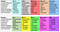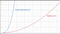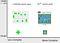# More facets of top-down puzzle game design

Presenting a correlation between solving methods and mathematical complexity in puzzles/problems.

## TL;DR

The classes of problems introduced in the previous article are further defined. More factors that relate to puzzles are suggested. The essence of a problem is related to mathematical analysis and how people perceive problems according to psychology. Summing it all up in a fairly cohesive model.

# Introduction

This article follows up on A top down approach to Puzzles in games. The previous article is not required to understand this one, but certainly helps in understanding the concepts discussed. The definitions in this article are the same as the predecessor: a puzzle is a type of problem with only a single solution. A problem, in turn, is a situation where there is some need to be fulfilled. Problems can either be continuous or finite: some have a definitive ‘begin’ and ‘end’ — ‘states’ if you like — and some don’t.

Heuristics: ‘fuzzy’ problem solving methods that use logic and guesses to solve a given instance of a general problem.

Lateral thinking: taking a step back from a problem and evaluating the goal, using creativity or coming up with novel approaches. Related but not the same as ‘out of the box thinking’

Deduction: solving a problem by ‘pure logic’: given a situation and it’s rules, combining those to arrive at a solid solution to the problem.

Deterministic: A situation that does not include random elements and is therefore fully ‘determinable’; deduction can be applied to predict any future of the situation.

# The factors within problems

What defines a problem from the player points of view? This question is important for both developers and players/reviewers/researchers. The factors I present here are based on personal views, loosely based on my personal gaming history, the Wikipedia article on problems, Social/Cognitive psychology and Algorithmic theory.

They are as follows:

• Penalties/rewards
• Instance size (e.g. small maze vs large maze)
• Inherent complexity (e.g. math crossword vs nonogram)
• Limited resources (e.g. time)
• Player knowledge
• Amount of solutions/methods
• Explorative vs clear goal

These are all broad factors, either hard to put an exact number on or a spectrum. The three last factors are slightly more fuzzy and require explanation to convey properly:

Player knowledge’ is about both what the player knew going into the game (really hard to measure) and what game has taught the player already. For instance, a player might have already learned the right-hand rule and traverses a maze more easily than the average player. In story driven games with decision points an experienced player could be more apt at ignoring choices that lead to ‘bad ends’.

Solutions/methods’ is about the ways a player can tackle a problem. This holds a complex relation to player knowledge as well. Well known is the choice between ‘high-risk, high-reward’ actions and ‘safe, low reward’ actions. In situations with this choice there are two methods. Many math oriented have only a single solution. Then moving towards highly complex games such as the Total War series there are many approaches to winning the game.

The last factor requiring explanation is ‘Explorative vs clear goal’. There seems to be a spectrum where at one side there are games that let the player do whatever. The other side is a clearly defined goal for players. In individual problems this pops up as well. When players get stuck on a Zelda Dungeon, it is not uncommon that the goal became more vague. Games like Sim City lack a clear goal and even border on not being a game anymore. Beyond those are simulators. A traditional action game has a very clear goal, often supported by a story. Between these extremes there are simulator-like games, Civilization as a notable example. A victory is achievable, but not the only way to have fun with the game. Moreover, a victory can be achieved through many methods and requires exploration of the mechanics to execute.

# Relation between problem factors and classes

The following table shows my proposal to how the classes of problems relate to factors of problems in general.Values for every factor differ in unit. ‘~variable’ indicates the class has no specific bias towards a value.

This table is to be interpreted as a handbook or guide, but not a step-by-step one, nor an exact theory.

# Zooming in on factors

Some factors have already been thoroughly discussed in the Game Design discourse. A well-known concept is the introduction of a mechanic and building it up so the player can master it easily. This handles player knowledge and is certainly applicable to puzzles/problems as seen in the Legend of Zelda series. Ernest Adams and Joris Dormans in their Game Mechanics book (2012) also addressed limited resources, albeit without explicitly applying to puzzles.

In the remainder of this article, we will be zooming in on instance size, complexity and and how players classifying how players approach problems.

The remaining factors (Explorative vs clear goal, Penalties/rewards) might be addressed in a future article. These factors link only loosely to the others, but strongly to each other.

# A short foray into algorithmic complexities

TL;DR: problems can be assigned a difficulty/complexity rating through complexity analysis.

In mathematics and computational (software) theory, there exists a concept about ‘classes’ of problem difficulties/complexity. One of the broadest and most famous is Computational complexity theory (CCT). In short it puts problems in categories based on how much time it would take to solve a non-trivial variant.

Let’s illustrate complexity in games: A friendly AI character needs to find a path from its current location to the player, in a 2D world. As any good AI does it will take the shortest path ;). For the shortest path there are a few algorithms to employ: for illustration we will compare Dijkstra’s Algorithm with Floyd-Warshall. Algorithms in the current context means ‘a method of solving’. Compare it with either asking people on the street for directions or using a map to get to some destination. Both approaches could be seen as algorithms for the problem of getting there.

In our example, the external effect for the player is the same: the AI walking towards them using the shortest path. Internally this algorithm choice matters a lot. The length of computing both algorithms mainly depends on one thing: the total amount of cells in the 2D world grid. Let us call this amount ’n’. For Dijkstra the order of time it takes is n² while Floyd-Warshall takes n³. Both exhibit stark growth as the game world would grow, but Floyd-Warshall is overall slower in being computed. It is these growth rates that are so essential to complexity.As the instance size of a problem increases, duration of solving increases. Some methods ‘scale’ worse than others.

This same comparison could be drawn in our previous scenario: in a complex city when your destination is, close it might be less time-consuming to ask someone for directions. Simplifying greatly, it is this analysis, and rating the performance, that is Computational Complexity Theory (CCT).

How does this relate to problems in general and problems/puzzles in games?Most, if not all, cognitive real life problems have a mathematical equivalent. This applies even more to videogames, that are already build on mathematical computer foundations. The Legend of Zelda: Oracles of Ages/Seasons have a notorious puzzle that is exactly the same as a ‘hard’ math problem. Unlike our shortest path example from before, solving this problem with a computer takes exponential time (some constant to the power of the amount of ‘cells’). Even the fastest method listed in this paper is still exponential. A puzzle the size of 40 tiles will take about 7700 solving steps to ensure a valid solution. Players struggle with these kinds of puzzles, so there seems to be a correlation…

The logical conclusion would be to directly apply CCT to game problems. That was my thought, at any rate. However, human brains and computers work differently. In our daily lives we solve problems such as the Shortest Path seen before. Attempting to divide the real world into cells using a computer, is a highly complex problem. Thusly, problems that in CCT would take days for a small computer can be solved by humans in the blink of an eye. Computers are simply too precise, human brains don’t require precision on the atom level. This does not mean that CCT is useless, just that playtesting trumps insight gained from CCT.

Instance size vs complexity becomes the interesting question. Going back to the Zelda Tile puzzles: why are they still manageable by even kids? Or look at Sokoban, which was proven to be really hard as well (here and here) The answer lies in instance size. What CCT tells us is that some aspect of the puzzle/problem, namely its ‘size’ dictates for the most part how long it will take to solve. While all Sokoban puzzles lie in the same ‘complexity’, the individual puzzles get larger and therefore take more time to solve.comparing the Lights Out problem with TLOZ Hamilton Path problem, top shows the predicted solving steps required for problems with the size of a 1000 ‘cells’

Let us assume that humans have three main methods of solving problems:

Guessing, Lateral thinking and Deduction. What humans use when, depends on the situation and prior knowledge. Being lost in a location is often solved mainly through guesses (how it feels is a different matter). Philosophers use Lateral thinking to question assumptions. When making a budget plan, Deduction is used.

This triad, when concerning games, form a spectrum. Clearly, between Guessing and Deduction there must be a space for Heuristics. Most what humans do is educated guessing i.e. Heuristics. Between Guessing and Lateral thinking there exists a space where riddles are solved. This is the scenario of the lost wanderer, walking in circles in a wood but then guessing a new direction after laterally realizing the circle. Then there is the complete opposite of Guessing: Deterministic solving. This area between Lateral and Deductive is where math and paradoxes rest. Real life is often too random and complex to be approached deductively, the models we make try to offer this space. For instance, models about materials are used to come to an optimally safe building, following logic.

What do players use in what scenario then? How does this apply to puzzles/problems when we have already defined factors?

The ‘nature’ or context of the problem defines in part how players react. A strategy game with many random elements will leave a player guessing. Players feeling stuck might resort to Lateral thinking. Deduction will be applied when solving a nonogram. The ‘nature’ of the problem defines how most players will interact with it. The instance size plays a role in that for small sizes players will tend to simply guess their way to victory.

# Condensed model

Using all of the previous knowledge we can create various models that can aid in deciding how problems should be implemented. At the same time analysists can use these to compare problems with.

This article will present one such model by condensing the problem-solving triad together with problem complexity, instance size and solving methods. In addition it marks the location of the problem classes (introduced in the previous article).

First, to condense the problem complexity and method into layers:

• Unit problems (puzzles)
• Normal problems, allow multiple solutions and/or methods
• Optimization problems, have a fuzzy goal and therefore even more ‘solutions’ then regular problems
• Wicked problems, that entails usually continuous problems without any true solutions. Multilemmas start playing a role and the approaches are numerous up to countable infinity.

Second, all these groups of problems contain all the types of the problem-solving triad. There are riddles with many answers, mathematical optimization problems and even heuristics with a single outcome.

Finally we populate this model with the problem classes. This shows the bias a class has for a certain complexity and solving method.

# Conclusion

We discussed a set of factors that defines problems in how they feel to a player. An overview of all the problem classes in relation to said factors was shown. Zooming in on complexity of problems, the adjacent Computation Complexity Theory was discussed. This gives us a theoretical direction as to why certain problems are easier than others. Next, the triad of problem-solving was presented. Deduction, Lateral thinking and Guessing lay on a spectrum with special intersections between them. All these models and hypotheses were combined into a single graph.